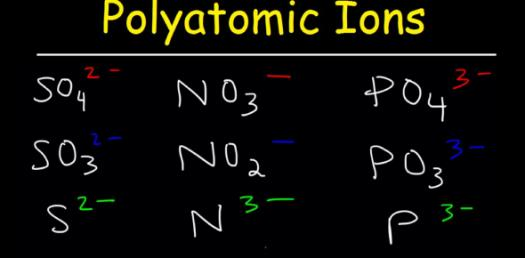# Names And Charges Of Ions: Test Your Knowledge! Trivia Quiz

45 Questions | Attempts: 2018SettingsIn the chemistry class, students learn about ions and that they usually have the same number of protons and neutrons. We also got to know some of the Ions, and in this trivia quiz, you will get to test your knowledge about names and charges of Ions. Think you can tackle it? Do give it a try and find out!

• 1.
The charge of beryllium is:
• A.

+2

• B.

-2

• C.

+1

• D.

-3

• E.

+3

• 2.
The charge of hydrogen is:
• A.

+2

• B.

-1

• C.

+1

• D.

-2

• E.

+3

• 3.
The charge of silver is:
• A.

+2

• B.

+1 and +2

• C.

-2

• D.

+1

• 4.
The charge of aluminum is:
• A.

+3

• B.

+2

• C.

+1

• D.

+4

• E.

-2

• 5.
The charge of carbonate is:
• A.

- 1

• B.

-3

• C.

-2

• D.

+3

• 6.
The symbol for zinc is
• A.

Zn

• B.

Z

• C.

Zi

• D.

Zc

• 7.
• A.

Pb

• B.

Ld

• C.

Le

• D.

La

• E.

Xe

• 8.
The symbol for ammonia is:
• A.

Am

• B.

A

• C.

An

• D.

NH4

• E.

NH2

• 9.
The charge for Li is:
• A.

+1

• B.

-1

• C.

+2

• D.

-2

• E.

+1 and +2

• 10.
The  symbol for tin is:
• A.

Sn

• B.

Tn

• C.

S

• D.

T

• E.

Ti

• 11.
B is what element?
• A.

Beryllium

• B.

Borinium

• C.

Barium

• D.

Bromide

• E.

Boron

• 12.
The symbol for strontium is
• A.

St

• B.

Sr

• C.

S

• D.

SO3

• E.

Sm

• 13.
The symbol for sulfide is
• A.

S

• B.

SO3

• C.

SO4

• D.

Sn

• 14.
Cl is the symbol for _____.
• 15.
The symbol for iron is____.
• 16.
C2H3O2 is the chemical formula for _________.
• 17.
The charge of iron is:
• A.

+1

• B.

+2

• C.

+3

• D.

+2 and +3

• E.

+1 and 3

• 18.
The symbol F represents ________.
• 19.
What is the name of NO2?
• 20.
What is the name of OH?
• 21.
The symbol for sodium is ____.
• 22.
The symbol for potassium is _______.
• 23.
The name for ClO4 is ____.
• 24.
The name for PO3 is ___.
• 25.
The charge of phosphate is:
• A.

-4

• B.

-3

• C.

-2

• D.

+3

• E.

+2

## Related TopicsBack to top
×

Wait!
Here's an interesting quiz for you.